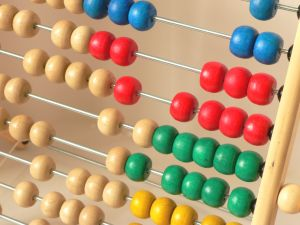Article

# Top Maths games for children

Elena Santos

PublishedI must admit I’m hopeless at maths. I’ve had a bad relationship with numbers since childhood: I remember doing my maths homework with a calculator beside me because I wouldn’t learn those darned multiplication tables. This is why I’m worried about teaching maths to kids, because I don’t want them to need a calculator to check their change every time they go shopping, just like me. As we all know, the best method to teach kids is playing games, so here’s a collection of free math/maths games that will help younger PC users develop their numeric skills.

• Math Games Level 1 – Basic maths for the youngest kids
• Math Niinja – Math exercises with 8 difficulty levels and progression logs
• Math Practice – Test your maths skills with this easy quiz
• Math-a-Maze – Find your way through a maze of numbers and maths operators
• TuxMath – An arcade game to be played with maths problems
• MathQuiz – Practice basic arithmetics with animated cartoons